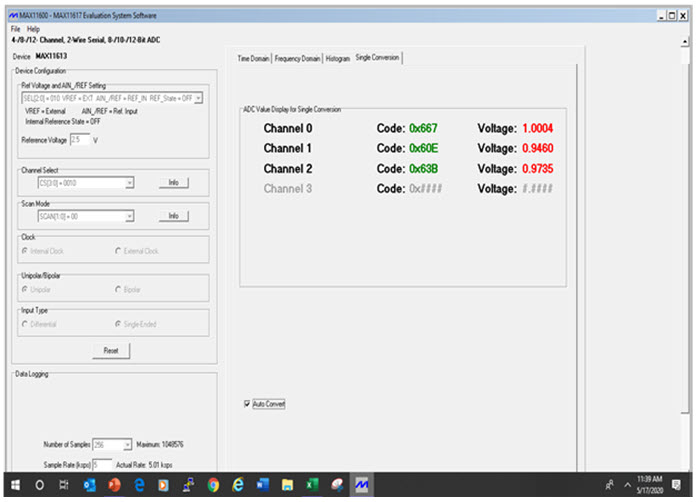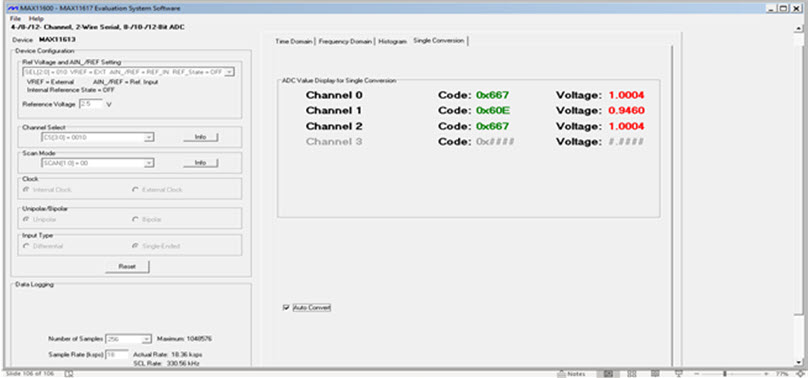# How to Improve ADC Measurement Accuracy with High-Input Source Impedance

### 要約

This application note discusses methods to use the MAX11613 ADC with high-input source impedance for accuracy.

### Introduction

Unbuffered ADCs are commonly used due to their simplicity of design. However, these ADCs have limited acquisition time and require input signals to settle within the allocated time. These ADCs must track their input signals for intervals longer than the input signal's settling time to achieve accurate conversion results. Hence, the need for low-input source impedance.

Successive-approximation-register (SAR) ADCs are frequently chosen for medium-to-high-resolution applications with sample rates under 5 megasamples per second (Msps). The resolution for SAR ADCs typically ranges from 8 to 20 bits. They provide low power consumption as well as a small form factor. These features make the ADCs ideal for a wide variety of applications such as portable/battery-powered instruments, pen digitizers, industrial controls, and data/signal acquisition.

The SAR ADC basically implements a binary search algorithm. Therefore, while the internal circuitry may be running at several megahertz (MHz), the ADC sample rate is a fraction of the number due to the successive-approximation algorithm.

The basic architecture of a SAR ADC is quite simple (Figure 1) though there are many variations to implement it. The analog-input voltage (VIN) is on a track/hold. The N-bit register is first set to midscale (100...00, where the most significant byte (MSB) is set to 1) to implement the binary search algorithm. This forces the DAC output (VDAC) to be VREF/2, where VREF is the reference voltage to the ADC. A comparison is done to determine if the VIN is less or greater than the VDAC. The comparator output is a logic-high or 1 and the MSB of the N-bit register remains 1 if the VIN is greater than the VDAC. Conversely, the comparator output is a logic-low and the MSB of the register is logic 0 if the VIN is less than VDAC. The SAR control logic then moves to the next bit that is down, forces it high, and does another comparison. The sequence continues all the way down to the least significant byte (LSB). The conversion is complete and the N-bit digital word is available in the register.Figure 1. Simplified N-bit SAR ADC architecture.

### MAX11163 Equivalent Input Circuit

The MAX11613 analog-input architecture contains an analog-input multiplexer (mux), a fully differential track-and-hold (T/H) capacitor, T/H switches, a comparator, and a fully differential switched capacitive digital-to-analog converter (DAC) (Figure 2). The analog-input multiplexer connects CT/H between the analog input in the single-ended mode, and to the + and – analog inputs in the differential mode.Figure 2. MAX11613 equivalent input circuit.

The T/H switches are in the track position and CT/H charges to the analog-input signal during the acquisition interval. The T/H switches move to the hold position at the end of the acquisition interval, retaining the charge on CT/H as a stable sample of the input signal. The switched capacitive DAC adjusts to restore the comparator input voltage to 0V within the limits of a 12-bit resolution during the conversion interval. This action requires 12 conversion clock cycles. It is equivalent to transferring a charge of 11pF x (VIN+ - VIN-) from CT/H to the binary-weighted capacitive DAC, forming a digital representation of the analog-input signal.

The sampling accuracy can get compromised if the source impedance is high (> 1.5k?), which causes the settling time of the input signal to be longer than the given acquisition time. The following experiments were carried out with different source impedance values.

### Experiments

The MAX11163 was configured to measure data at three channels: CH0, CH1, and CH1 to see the effects of the high-impedance source on the measured accuracy. Figure 3 shows the data with three different source impedances. CH0 has a low 50? source impedance. CH1 has a 10k? source impedance with an additional 100pF capacitor connected from the analog input to the ground. CH1 has a 10k? source impedance. The measured data of CH0 (1.0004V) has an error of approximately -0.04% with an input voltage of 1.0000V. The measured data of CH1 (0.9460) has an error of approximately 5.5%. The MAX11613 measured the input voltage of 1.0000V as 0.9735 without the additional 100pF capacitance at the input. There was an error of approximately 2.69%.Figure 3. MAX11613 captured data from 1.0000V input voltage with different source impedances.

### Accuracy Improvements

Three methods can be used to minimize sampling errors with higher source impedances.

### Method 1. Buffer Amplifier

The MAX44244 provides a low-output impedance when configured as a buffer amplifier (Figure 4) for the MAX11613 to capture the signal accurately. The MAX44244 also offers a very low offset of 2µV, making it ideal to pair with the MAX11163 for high-accuracy measurement with high-input source impedance up to 1MHz. The MAX44244 is the best choice as a buffer amplifier for higher input frequency up to 10MHz.Figure 4. MAX44242/MAX44244 buffer amplifier.

The results show all the three channels have low-accuracy errors of only -0.04% with the buffer amplifiers connected after the two channels with high-input impedance.Figure 5. Data with buffer amplifiers following CH1 and CH1 (high-input source impedance).

### Method 2. High CEXT Capacitance

The measured accuracy error of the device improved tremendously to -0.04% as if the input had a low-impedance source (Figure 6) by installing a high external capacitance at channel 2 (CEXT = 0.1µF) at the analog input of the MAX11613 ADC. The high capacitance of 0.1µF can store sufficient charge to quickly charge the internal sampling capacitor (CIN). Therefore, the settling time is only RIN × CIN instead of the much longer (RSOURCE + RIN) × CIN. Thus, the accuracy improved significantly.Figure 6. Data with 1.0000V input. CH0 source impedance is 50?. CH1 source impedance is 10k? with 100pF to the ground. CH1 source impedance is 10k? with 0.1µF to the ground.

This second method to improve measurement accuracy is suitable for a DC or near-DC input signal only due to the high input capacitor (CEXT) of at least 0.1µF. This 0.1µF capacitor provides a cut-off frequency of 1/(2 × 3.1416 × 10k? × 0.1µF) = 159Hz coupled with the 10k? input source impedance.

### Method 3. Lower External SCL Serial Clock Frequency

If the analog-input source impedance is high, the acquisition time constant lengthens and more time must be allowed between conversions. The third method to achieve high conversion accuracy is to lower the external SCL serial clock frequency to provide the needed additional conversion time. Figures 7 and 8 depict the accuracy errors of 1.37% with SCL= 400kHz and 0.27% with SCL= 90kHz, respectively.Figure 7. RSOURCE CH0 = 50?, CH1 = 10k?/1000pF, VIN = 1.0000V, VADC(CH0) = 1.0004V (Error = -0.4%), VADC(CH1) = 0.9863V (Error = 1.37%), fSCL = 400kHz.Figure 8. RSOURCE CH0 = 50?, CH1 = 10k?/1000pF, VIN = 1.0004V, VADC(CH0) = 1.0004V (Error=0%), VADC(CH1) = 0.9973V (Error = 0.27%), fSCL= 90kHz.

### Conclusion

This document illustrates three methods to improve the measured accuracy of the MAX11163 with high-input source impedance. The buffer amplifier method is the best solution for a high input frequency of up to 5MHz using the MAX44242. The MAX44244 can provide a lower accuracy error due to its very low offset voltage of only 2µV for a lower input frequency of up to 1MHz. A simpler and lower cost solution for a DC or low-input frequency of up to 100Hz is to use a high-value capacitor of 0.1µF connected from the analog input to the ground to provide low-impedance charging to the internal sampling capacitor (CT/H). The least expensive method to improve the conversion accuracy is to simply reduce the external SCL serial clock frequency, which can be accomplished by a software change.